Name:    3rd Grade Math Proficient Test 8

Multiple Choice
Identify the choice that best completes the statement or answers the question.

1.

Number Sense and Numeration – Whole Numbers – RIT 191 – 200

Which number is greater than eight thousand nine hundred nine?
 a. 8,900 d. 8,800 b. 8.908 e. 80,000 c. 8,000

2.How many dozen doughnuts?
 a. 3 d. 2 1/2 b. 24 e. 4 c. 2

3.

Round 86,463 to the nearest ten thousand.
 a. 80,000 d. 90,000 b. 86,000 e. 86,400 c. 86,460

4.

Which are only prime numbers?
 a. 1, 3, 9, 12 d. 11, 17, 67, 19 b. 8, 16, 24, 36 e. 7, 49, 63, 77 c. 13, 23, 45, 68

5.

In which set are the numbers divisible by 2?
 a. 8, 10, 15 d. 20, 30, 45 b. 23, 37, 46 e. 9, 17, 23 c. 64, 90, 96

6.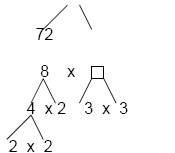What is the missing factor in this factor tree?
 a. 6 d. 2 b. 8 e. 12 c. 9

7.

54 = 9 x 6
What is another way of naming 54?
 a. 2 x 3 x 9 d. 3 x 2 x 4 b. 3 x 3 x 2 x 2 e. 3 x 16 c. 26 x 2

8.

What is the LCM (least common multiple) of 3 and 12?
 a. 3 d. 36 b. 24 e. 4 c. 12

9.

What is 0.75 as a fraction?
 a. 1/4 d. 7/10 b. 1/2 e. 3/4 c. 2/3

10.

Round to the nearest tenth: 3.276
 a. 3.3 d. 3.28 b. 3.2 e. 3.27 c. 0.3

11.

2.378 x 100 =
 a. 0.2378 d. 237.8 b. 2.378 e. 23.78 c. 2378

12.

Number Sense and Numeration – Exponents – RIT 201 – 210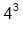a. 12 d. 256 b. 16 e. 4 c. 64

13.

Number Sense and Numeration – Fractions – RIT 201 –210

What is the simplest form of 15/20?
 a. 15/20 d. 1 5/20 b. 3/4 e. 5/20 c. 2/3

14.

____/18 = 2/3
 a. 9 d. 6 b. 10 e. 12 c. 15

15.

Identify the fraction the dot represents between 0 and 1.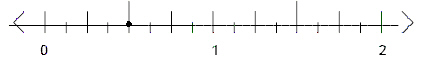a. 1/3 d. 4/8 b. 5/8 e. 5/4 c. 5

16.

Number Sense and Numeration – Ordering, Equalities and Inequalities –
RIT 201 – 210

Put the following numbers in order from least to greatest:
401, 210, 315, 211, 314, 423
 a. 210; 211; 314; 315; 401; 423 d. 210; 401; 211; 423; 314; 315 b. 423; 401; 315; 314; 211; 210 e. 211; 210; 315; 314; 423; 401 c. 401; 210; 211; 314; 315; 423

17.

Select the correct symbol for the box:
2,347,228,0012,347,598,001
 a.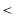d.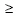b.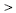e.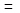c.18.

Put the following numbers in order from least to greatest:
1/2, 0, -2/3, -3, 5
 a. 0; 1/2; -2/3; -3; 5 d. 5; -3; 1/2; -2/3; 0 b. –3; -2/3; 0; 1/2; 5 e. 0; -2/3; 1/2; -3; 5 c. 5; -3; -2/3; 1/2; 0

19.

Translate the following sentence into an equation:
“Twice a number, plus five, equals twenty-five.”
 a. 2(x + 5) = 25 d. x + 5 = 25 b. 2x – 5 = 25 e. x2 + 5 = 25 c. 2x + 5 = 25

20.

Put the following exponential values in order from least to greatest: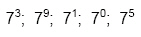a.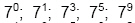d.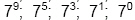b.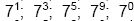e.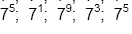c.21.

Number Sense and Numeration – Percents – RIT 201 – 210

What is 60% of 20?
 a. 120 d. 60 b. 12 e. 10 c. 80

22.

1/8 expressed as a percent is:
 a. 125% d. 1.25% b. 0.125% e. 12% c. 12.5%

23.

Number Sense and Numeration – Place Value – RIT 201 – 210

2,478,015,963
Which digit is in the ten-millions place?
 a. 4 d. 6 b. 7 e. 8 c. 1

24.

Which number represents, “five and twenty-three hundredths”?
 a. 5.230 d. 52.3 b. 5.23 e. 0.523 c. 523 hundredths

25.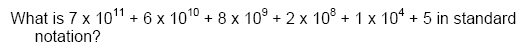a. 768,215 d. 7,682,015 b. 768,200,015 e. 768,200,010,005 c. 700,600,800,200,100,005